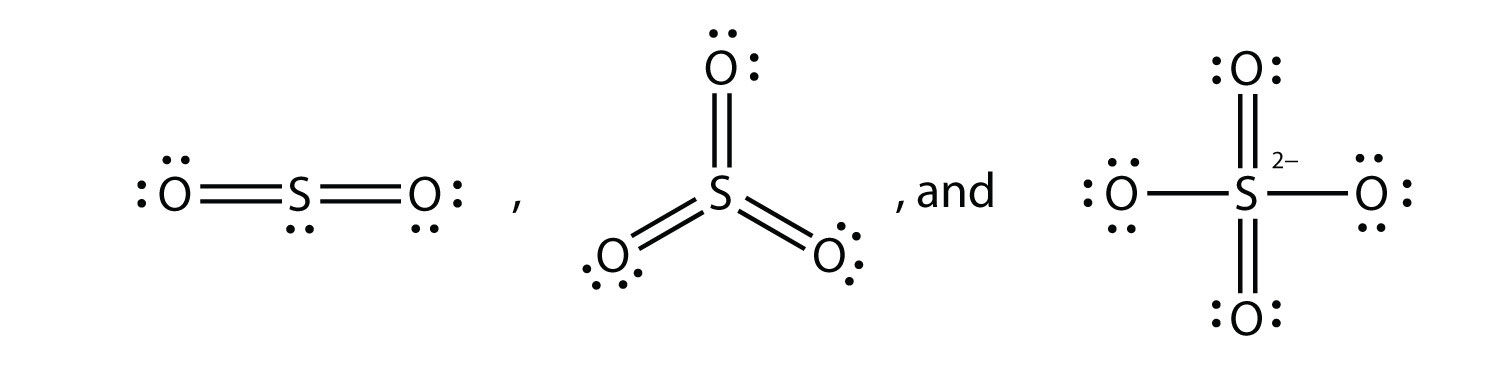This is “End-of-Chapter Material”, section 9.7 from the book Beginning Chemistry (v. 1.0). For details on it (including licensing), click here.

Has this book helped you? Consider passing it on:
Creative Commons supports free culture from music to education. Their licenses helped make this book available to you.
DonorsChoose.org helps people like you help teachers fund their classroom projects, from art supplies to books to calculators.

## 9.7 End-of-Chapter Material

1. Explain why iron and copper have the same Lewis electron dot diagram when they have different numbers of electrons.

2. Name two ions with the same Lewis electron dot diagram as the Cl ion.

3. Based on the known trends, what ionic compound from the first column of the periodic table and the next-to-last column of the periodic table should have the highest lattice energy?

4. Based on the known trends, what ionic compound from the first column of the periodic table and the next-to-last column of the periodic table should have the lowest lattice energy?

5. P2 is not a stable form of phosphorus, but if it were, what would be its likely Lewis electron dot diagram?

6. Se2 is not a stable form of selenium, but if it were, what would be its likely Lewis electron dot diagram?

7. What are the Lewis electron dot diagrams of SO2, SO3, and SO42−?

8. What are the Lewis electron dot diagrams of PO33− and PO43−?

9. Which bond do you expect to be more polar—an O–H bond or an N–H bond?

10. Which bond do you expect to be more polar—an O–F bond or an S–O bond?

11. Use bond energies to estimate the energy change of this reaction.

C3H8 + 5O2 → 3CO2 + 4H2O
12. Use bond energies to estimate the energy change of this reaction.

N2H4 + O2 → N2 + 2H2O
13. Ethylene (C2H4) has two central atoms. Determine the geometry around each central atom and the shape of the overall molecule.

14. Hydrogen peroxide (H2O2) has two central atoms. Determine the geometry around each central atom and the shape of the overall molecule.

1. Iron has d electrons that typically are not shown on Lewis electron dot diagrams.

2. LiF

3. It would be like N2:4.5. an O–H bond

6. −2,000 kJ

7. trigonal planar about both central C atoms